• 相对论公式傅里叶级数展开的波函数离散解的本质分析探讨，苏力宏，，本论文依据相对论方程的傅里叶级数表达式的波函数方程，对于引力场、量子力学电磁方程进行了新的解释应用，根据物质波理论对
• 首先，傅里叶分析是指把一个周期...傅里叶级数 三角函数系的正交性 三角函数系：{1,sinx,cosx,sin2x,cos2x,…,sinnx,cosnx,…}，它由无数个sinnxcosnx组成，其中n=0,1,2,…。 正交性： ∫−ππsin⁡nxcos⁡mxdx=0，
首先，傅里叶分析是指把一个周期或非周期函数展开成一个个三角函数的叠加，如果是对其还没有基本概念的，可以看看傅里叶分析之掐死教程，这篇文章不依赖数学公式却又十分透彻地讲述了傅里叶分析的基本概念，十分值得一读。但如果先深入探讨其中的数学由来，接下来会讲述详细的数学推导。
傅里叶级数
三角函数系的正交性
三角函数系：{1,sinx,cosx,sin2x,cos2x,…,sinnx,cosnx,…}，它由无数个sinnx和cosnx组成，其中n=0,1,2,…。
正交性：
$\int^\pi_{-\pi}\sin nx \cos mx dx = 0$
$\int^\pi_{-\pi}\cos nx \cos mx dx = 0，n\neq m$
$\int^\pi_{-\pi}\sin nx \sin mx dx = 0，n\neq m$
当向量点乘等于0：
$\vec a \cdot \vec b = 0$
则两个向量正交。
拓展到函数中，两个函数相乘，原本点乘的加和变成取积分，则：
$\int^{x_1}_{x_2}f(x) g(x) dx = 0$
称为两个函数正交。
证明其正交性，可以用积化和差公式：
$\int^\pi_{-\pi}\cos nx \cos mx dx = \frac{1}{2}\int^\pi_{-\pi}[cos(n-m)x+cos(n+m)x]dx =0$
其他情况同理。
周期为2π的函数展开
因此当一个函数f(x)周期为2π时，可以展开成
$f(x) = \sum^\infty_{n=0}a_ncosnx + \sum^\infty_{n=0}b_nsinnx = \frac 1 2 a_0 + \sum^\infty_{n=1}({a_ncosnx + b_nsinnx})$
对两边取积分，由于三角函数的正交性
$\int_{-\pi}^\pi f(x)dx = \frac1 2\int_{-\pi}^\pi a_0dx = \pi a_0$
$a_0 = \frac 1 {\pi}\int_{-\pi}^\pi f(x)dx$
原式乘以cos mx 再对两边取积分可得：
$\int^\pi_{-\pi}f(x) \cos mx dx = \sum^\infty_{n=1}\int^\pi_{-\pi} a_ncosnx cosmx dx = \int^\pi_{-\pi} a_ncos^2 nx dx = a_n\pi$
$a_n = \frac 1 \pi \int^\pi_{-\pi}f(x) \cos nx dx$
同理，两边同乘sinmx再取积分可得：
$b_n = \frac 1 \pi \int^\pi_{-\pi}f(x) \sin nx dx$
周期为2L的函数展开
利用换元的方法，令：$x = \frac \pi L t$ ，即$t = \frac L \pi x$，可得：
$f(t) = \frac {a_0} 2 + \sum^\infty_{n=1}({a_ncos \frac{n\pi}{L}t + b_nsin\frac{n\pi}{L}t})$
$a_0 = \frac 1 {L}\int_{-L}^L f(t)dt$
$a_n = \frac 1 L \int^L_{-L}f(x) \cos nx dx$
$b_n = \frac 1 L \int^L_{-L}f(x) \sin nx dx$
在工程中t总是从0开始，周期T=2L，ω = $\frac \pi L = \frac 2\pi T$，此时：
$f(t) = \frac {a_0} 2 + \sum^\infty_{n=1}({a_ncosωt + b_nsinωt})$
$a_0 = \frac 2 {T}\int_{0}^T f(t)dt$
$a_n = \frac 2 T \int^T_{0}f(x) \cos nx dx$
$b_n = \frac 2 T \int^T_{0}f(x) \sin nx dx$
傅里叶级数的复数表达形式
欧拉公式：$e^{iθ} = cosθ + isinθ$
可得：
$cosθ = \frac 1 2 (e^{iθ}+e^{-iθ})$
$sinθ = \frac 1 2 i(e^{iθ}-e^{-iθ})$
把这两条式子代入f(t)的式子中可得：
$f(t) = \frac {a_0} 2 + \sum_{n=1}^\infty \frac {a_n-ib_n} 2 e^{in\omega t}+ \sum_{n=1}^\infty \frac {a_n+ib_n} 2 e^{-in\omega t} = \sum_{n=0}^0 \frac {a_0} 2 e^{in\omega t} + \sum_{n=1}^\infty \frac {a_n-ib_n} 2 e^{in\omega t}+ \sum_{n=-1}^{-\infty} \frac {a_{-n}+ib_{-n}} 2 e^{in\omega t} = \sum_{-\infty}^\infty C_n e^{in\omega t}$
当n = 0时，
$C_n = \frac {a_0} 2 = \frac 1 {T}\int_{0}^T f(t)dt$
当n > 0时，
$C_n = \frac {a_n-ib_n} 2 = \frac 1 2 (\frac 2 T \int_0^T f(t)cos{n\omega t} - i\frac 2 T \int_0^Tf(t)sin{n\omega t}) = \frac 1 T \int_0^T f(t)(cos{n\omega t} - isin{n\omega t}) dt = \frac 1 T \int_0^T f(t) e^{-in\omega t}dt$
当n < 0时，
$C_n = \frac {a_{-n}+ib_{-n}} 2 = \frac 1 T \int_0^T f(t)(cos{n\omega t} - isin{n\omega t}) dt = \frac 1 T \int_0^T f(t) e^{-in\omega t}dt$
因此得出结论，一个周期函数f(t)有f(t)=f(t+T)时：
$f(t) = \sum_{-\infty}^\infty C_n e^{in\omega t}$
$C_n = \frac 1 T \int_0^T f(t) e^{-in\omega t}dt$
傅里叶变换
对于$C_n$来说，它的值是一个复数，而nω是一个离散的值，那么可以在代表nω的轴上一个个特定的点上设一个平面，这个平面是一个复平面，它的长度和方向代表$C_n$：在工程上，横坐标为时间的波形图称为时域表达，而这幅图显示的是在各种不同频率下的值，称为频域表达，也是波形图的频谱，这就是从不同的角度看时间，每一种波形都对应一种频谱。不过很多时候的频谱都不是这种复平面三维的，我们会把$C_n$的幅度即模单独拿出来，就可以表示这个函数在不同频率下的强度了。当T增大时，ω数值减小，nω之间就挨得越近。T趋于无穷时会形成一条连续的曲线：由：
$f(t) = \sum_{-\infty}^\infty C_n e^{in\omega t}$
$C_n = \frac 1 T \int_0^T f(t) e^{-in\omega t}dt$
得到：
$f_T(t) = \sum_{n=-\infty}^{\infty}\frac 1 T \int_{-\frac T 2}^{\frac T 2} f_T(t) e^{-in\omega_0 t}dt e^{inω_0t}$
$f_T(t) = \sum_{n=-\infty}^{\infty}\frac {\Deltaω} {2\pi} \int_{-\frac T 2}^{\frac T 2} f_T(t) e^{-in\omega_0 t}dt e^{inω_0t}$
当$T \rightarrow \infty$：
$f(t) = \frac {1} {2\pi} \int_{-\infty}^{\infty} \int_{-\infty}^{\infty} f(t) e^{-i\omega t}dt e^{inωt}d\omega$
因此我们把中间的公式称为傅里叶变换(FT)：
$F(ω) = \int_{-\infty}^{\infty} f(t) e^{-i\omega t}dt$
通过这个函数可以表示在任何频率的情况下的三角函数的波形的振幅，这通常是一个复数a+bi，其中实数部分代表cos，虚数部分代表sin。而负频率没有现实意义，其振幅为正频率的共轭，只是为了在数学上的计算便利，实际上用$\sqrt{a^2+b^2}$表示其振幅。
外面套的公式称为傅里叶变换的逆变换(IFT)：
$f(t) = \frac {1} {2\pi} \int_{-\infty}^{\infty} F(ω) e^{iωt}d\omega$
傅里叶变换的所有内容讲解完毕了，傅里叶变换其实是一种特殊的拉普拉斯变换(s=iω)，遵循拉普拉斯变换的所有性质。


展开全文经验分享
• 傅里叶级数公式还是有点复杂的，以致很多人记不住。本文就讲授一种有利于记忆的方法，以作科普。向量对于傅里叶级数，我从向量开始讲。有人可能会问，这两者似乎没什么太大的联系？别急，且看我下面的讲述。三维...
傅里叶级数(变换)对于很多理工学科是非常重要的分析工具，比如电子学中，对电信号的时域、频域的变换。但傅里叶级数的公式还是有点复杂的，以致很多人记不住。本文就讲授一种有利于记忆的方法，以作科普。向量对于傅里叶级数，我从向量开始讲。有人可能会问，这两者似乎没什么太大的联系？别急，且看我下面的讲述。三维空间中的向量可以表示为：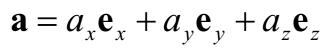式(1)上面黑体字的 ex、ey和 ez分别表示三维笛卡尔正交坐标系下 x、y和 z轴上的单位向量。只要学过高中数学，都知道向量的内积，也就是两个向量的点乘。向量的点乘有非常实际的物理意义，比如水平面上有一个滑块在力 F 的作用下，平移了位移 r，如果力的方向和位移r 不共线，那么力 F 对滑块所做的功为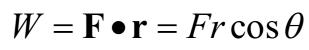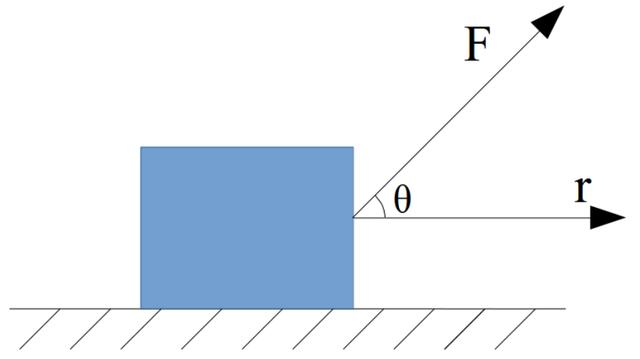图1 内积与做功图 1 内积与做功这里对内积作了简单的展开。那对三维空间中的任意两个向量的点乘，可表示为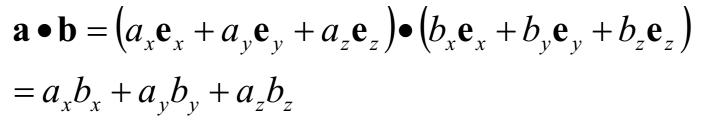式(2)如果两个向量的内积为零，那么就说这两个向量是相互正交的，也就是相互垂直。那么如果(2)式中的 b向量是 x轴上单位向量，那么有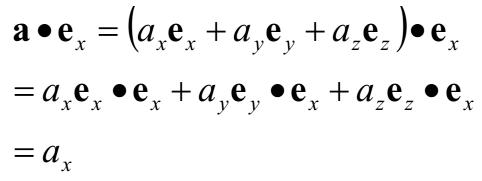式(3)类似的，可以分别得到 ay和 az。接下来，把向量的维数推广到任意正整数 N，N可以大于 3乃至趋于无穷大。而 N维的笛卡尔正交坐标系的每一个单位向量表示为 e1,e2,e3,...eN，这样就把(1)式改写为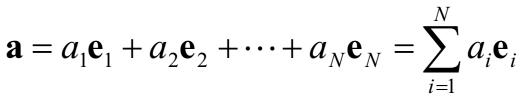式(4)则任意两个向量的内积为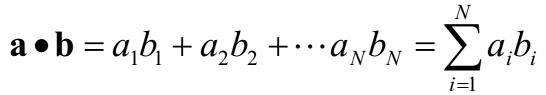式(5)而如果向量 b 是 N维笛卡尔坐标系上第 j个单位向量，那么根据(3)式的结论，可以求出向量 a 在第 j个坐标上的分量。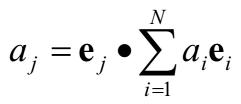式(6)因为有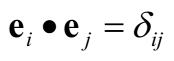其中δij称为克罗内克符号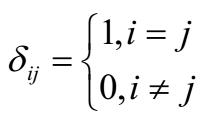也就是说如果整数 i=j，δij=1；如果 i≠j，δij=0.粗略地讲，求积分就是求和的推广，因此可以把上述结论推广到函数，也就是说把函数看成是一个向量，并且这个向量的维数是无穷的。函数设有定义在实数轴区间[a,b]上的黎曼可积函数 f(x)，g(x)，a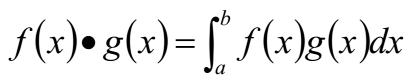式(7)那么怎么把(7)式与向量的内积联系起来？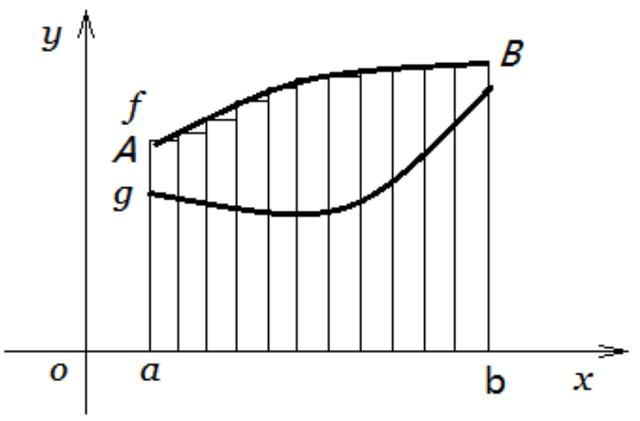图2 函数的黎曼分割在图 2中，将定义域[a,b]均匀等分为 N段，这样的分割称为黎曼分割。学过微积分的读者应该对上面这个图非常熟悉。函数 f(x)在定义域[a,b]上表示的曲线段，到 x轴[a,b]上的这一块不规则图形的面积可以近似为图中一块一块长方体面积的和，也就是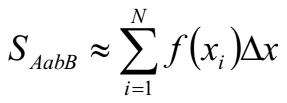式(8)设想一下，将 g(x)这段曲线以 x轴为中心轴旋转 90度，并作出如图 3所示的不规则柱状体。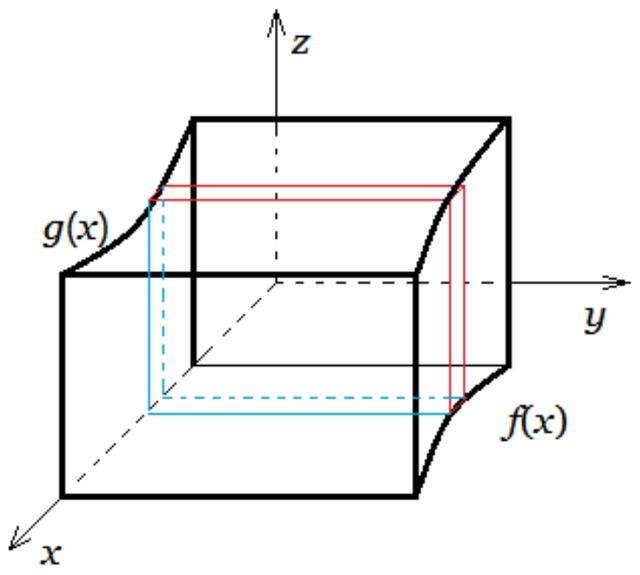图 3 函数内积的示意图这个柱状体的体积可以近似地表示为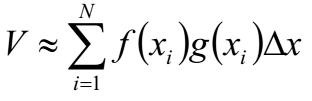式(9)观察(9)式与(5)式，在形式上只相差一个Δx.当分割趋于无穷大时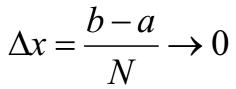此时Δx可以用微分元 dx表示，即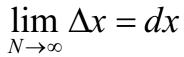于是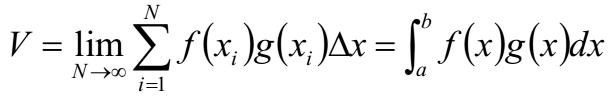式(10)这就是两个函数的内积的形象示意。如果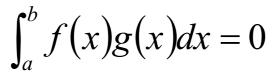则说函数 f(x)和 g(x)在定义域[a,b]上是相互正交的，表示为 f(x)⊥g(x).那么，这个"函数的内积"与傅里叶级数或傅里叶变换有什么关系呢？傅里叶级数(变换)对于一个基本周期为 2π的周期函数，其复数形式的傅里叶级数展开为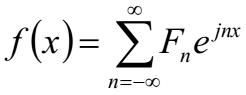式(11)系数的表达式为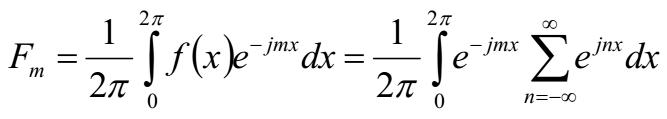式(12)其中 j是虚数单位，用 j表示虚数单位是电子学里的习惯，以与电流 i作区别。而 Fn是一个复数。可以发现，把函数视作向量后，(11)式与(6)式是很相似的，(12)式与(10)式很相似。是何道理呢？道理在于，既然把函数视作向量后，向量是可以用坐标表示的，这是(6)式的意义所在，而且这个坐标系最好是正交坐标系。那就要找出一个在定义域上的函数向量正交坐标系。设定义域[a,b]上有一组函数向量正交基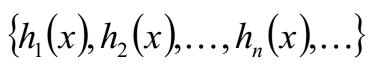式(13)这是一组无限维的向量基，这是与有限维空间坐标系的重要区别。则对于定义在[a,b]上的黎曼可积的实函数 f(x)可展开为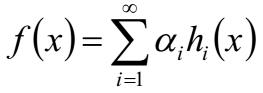式(14)其中αi是一个实数。根据向量的运算关系，有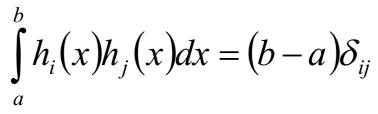式(15)这样，(14)式中的系数可表示为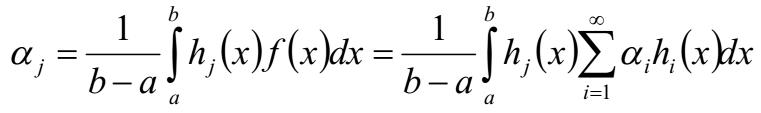式(16)这时(14)式又和(11)式对应起来了，而(16)式又和(12)式对应起来了。当然，实函数与复函数的情况是有区别的。在复数形式的傅里叶级数中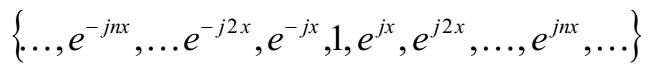式(17)正是定义域[0,2π]上的一组复正交坐标系。有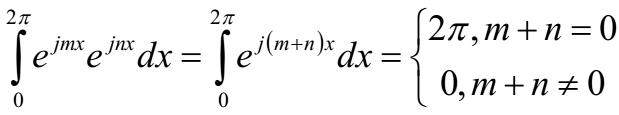上面(12)式与(16)式的区别在于，两个复函数求内积时，要对其中一个复函数求共轭。当函数为非周期函数时，傅里叶级数就扩展为傅里叶变换和傅里叶反变换。傅里叶变换中是将非周期信号看成是周期为无穷大的周期信号，因此替换(11)式中的 x为 2πt/T，对 T→∞求极限，而当 T→∞时，2π/T就变成了角频率的微分元。即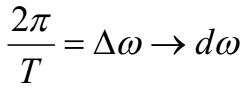而 nΔω可以写成ω，即ω=nΔω，n=ω/Δω于是改写(11)式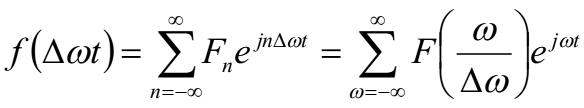式(18)显然，上面这个式子是不收敛的。为了确保其收敛，在左右两边同除以周期 T，也就是要关于周期求平均。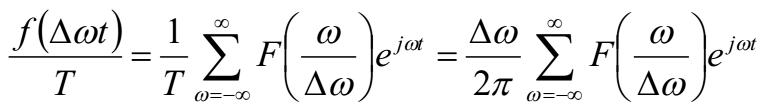式(19)需要注意的是，此时因为周期 T是常数，所以Δω也是个常数，但ω是变数。所以可以改写(19式)为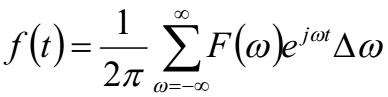式(20)现在开始对 T→∞求极限，求和变成积分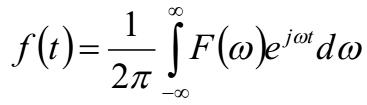式(21)这就是非周期函数的傅里叶反变换。到了这一步我们要求出系数方程。对于一个特定的ω0，对(21)式两边求积分。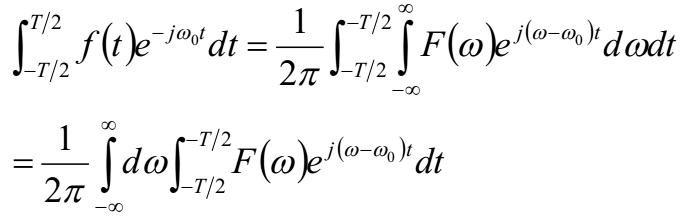式(22)对(22)式关于 T→∞求极限，并结合前面关于正交基的讨论，有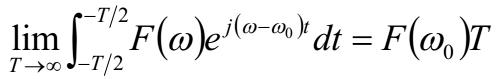进而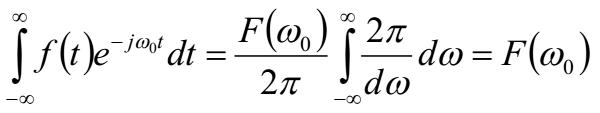又因为ω0是任意的，所以有傅里叶变换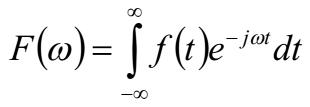式(23)总结与补充透过现象看本质。一个通常的(只说常见的函数，此文不作展开)可积函数是无限维函数向量空间上的一个向量。既然是向量就要符合向量的运算法则，而傅里叶级数展开与傅里叶变换正是要寻找此向量空间中的一组正交坐标基，这组坐标基的每一个坐标分量也是一个函数——正弦函数或复指数函数。在使用傅里叶级数展开和傅里叶变换时，需要注意以下几点：①如果向量所在的空间是刚性的，即一个向量在空间中作平移、旋转等运动时，向量不会被伸缩和扭曲。则向量的内积与坐标系无关。在(2)式中，是两个正交坐标系下的向量的内积，而(10)式中的函数向量内积是在仿射坐标系下的内积，这个内积与正交坐标系下求得的内积应该是相等的。也就是说对(11)式的函数作内积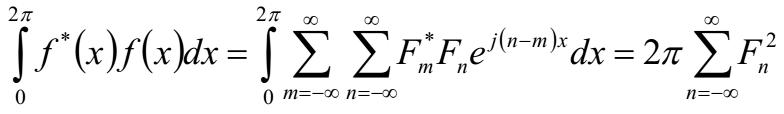式(24)②当函数是定义域为实数的复函数时，求函数内积的时候要对其中一个函数取共轭，如(24)式；③在(24)式右侧既然是一个无穷级数，就要考虑收敛性。也就是说 n→±∞时，Fn→0。而如果(23)式成立，要满足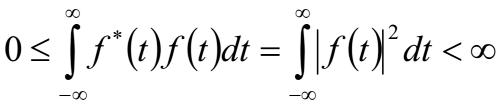如果 f(t)代表的物理量是电压或电流，则意味着在一段足够长的时间里，能量也是有限的，否则不能用傅里叶变换。声明：原创文章。未经允许，不得转载！
展开全文• 傅里叶级数和傅里叶变换基本定理三角函数的正交性欧拉公式傅里叶级数三角形式指数形式傅里叶变换傅里叶积分定理 最近在做音频处理相关的内容，所以打算对傅里叶的相关内容做一个小结。大学本科时学过复变函数，不过...
傅里叶级数和傅里叶变换基本定理三角函数的正交性欧拉公式傅里叶级数三角形式指数形式傅里叶变换傅里叶积分定理
最近在做音频处理相关的内容，所以打算对傅里叶的相关内容做一个小结。大学本科时学过复变函数，不过现在已经忘的差不多了，然后毕业后也经常会用到这个东西，无论是一维的信号还是二维的图像，所以就打算对此做一个理论推导。另外，在下文推导中全部假设所有函数都满足可进行傅里叶变换的条件假设，对于其他一些特殊函数，不在本文讨论范围之内。
基本定理
三角函数的正交性
对于三角函数组合 $1, sinx , cosx, sin2x, cos2x,...,sinnx,cosnx,...$中任意两个函数的乘积在$[-\pi,\pi]$上的积分都为0，即$\int\limits_{-\pi}^{\pi} {sin(x)}dx=0$    $\int\limits_{-\pi}^{\pi} {cos(x)}dx=0$ $\int\limits_{-\pi}^{\pi} {sin(mx)cos(nx)}dx=0$$\int\limits_{-\pi}^{\pi} {sin(mx)sin(nx)}dx=0$ $\int\limits_{-\pi}^{\pi} {cos(mx)cos(nx)}dx=0$
欧拉公式
$e^{ix}=cos(x) +isin(x)$
傅里叶级数
三角形式
对于一个周期为 $T$ 的函数，可以用一系列三角函数的叠加组成，令$\omega={2\pi \over T}$，则
$f(t) = {b_0 \over 2} + \sum \limits_{n=1}^{+\infty}{[a_n sin(n\omega t)+b_ncos(n\omega t)]}$
对上式在某个周期上进行积分可得$\int\limits_{{t_0}}^{{t_0} + T} {f(t)dt = } \int\limits_{{t_0}}^{{t_0} + T} { {b_0 \over 2}} dt + \int\limits_{{t_0}}^{{t_0} + T} \sum \limits_{n=1}^{+\infty} {[{a_n}\sin (n\omega t) + {b_n}\cos (n\omega t)]} dt$$= {b_0 \over 2}T + {{2\pi } \over T}\int\limits_{{x_0}}^{{x_0} + 2\pi } \sum \limits_{n=1}^{+\infty} {[{a_n}\sin (nx) + {b_n}\cos (nx)]} dx=b_0T$得到，$b_0={2 \over T} \int\limits_{{t_0}}^{{t_0} + T} {f(t)dt}$。
对上式两边同时乘以$sin(m\omega t)$，再在某个周期上进行积分可得$\int\limits_{{t_0}}^{{t_0} + T} {f(t)\sin (m\omega t)dt = } {a_0}\int\limits_{{t_0}}^{{t_0} + T} {\sin (m\omega t)} dt + \int\limits_{{t_0}}^{{t_0} + T} {\sum\limits_{n = 1}^{+\infty} {[{a_n}\sin (n\omega t)\sin (m\omega t ) + {b_n}\cos (n\omega t)\sin (m\omega t )]} } dt$$= {a_m}\int\limits_{{t_0}}^{{t_0} + T} {{{\sin }^2}(m\omega t)} dt = {{{a_m}} \over 2}\int\limits_{{t_0}}^{{t_0} + T} {[1-\cos (2m\omega t)} ]dt = {{{a_m}} \over 2}T$
得到$a_m ={2 \over T} \int\limits_{{t_0}}^{{t_0} + T} {f(t)\sin (m\omega t)dt}$
对上式两边同时乘以$cos(m\omega t)$，再在某个周期上进行积分可得$\int\limits_{{t_0}}^{{t_0} + T} {f(t)\cos (m\omega t)dt = } {a_0}\int\limits_{{t_0}}^{{t_0} + T} {\cos (m\omega t)} dt + \int\limits_{{t_0}}^{{t_0} + T} {\sum\limits_{n = 1}^{+\infty} {[{a_n}\sin (n\omega t)\cos (m\omega t ) + {b_n}\cos (n\omega t)\cos (m\omega t )]} } dt$$= {b_m}\int\limits_{{t_0}}^{{t_0} + T} {{{\cos }^2}(m\omega t)} dt = {{{b_m}} \over 2}\int\limits_{{t_0}}^{{t_0} + T} {[\cos (2m\omega t)} + 1]dt = {{{b_m}} \over 2}T$
得到$b_m ={2 \over T} \int\limits_{{t_0}}^{{t_0} + T} {f(t)\cos (m\omega t)dt}$
综上，可以得到傅里叶级数及其系数的形式，如下
$f(t) = {b_0 \over 2} + \sum \limits_{n=1}^{+\infty}{[a_n sin(n\omega t)+b_ncos(n\omega t)]}$$a_n ={2 \over T} \int\limits_{{t_0}}^{{t_0} + T} {f(t)\sin (n\omega t)dt}$ $b_n ={2 \over T} \int\limits_{{t_0}}^{{t_0} + T} {f(t)\cos (n\omega t)dt}$
指数形式
根据欧拉公式可以得到
$cos(x) = {{e^{ix} + e^{-ix}} \over 2}$ $sin(x) = {{e^{ix} - e^{-ix}} \over 2i}$ 代入傅里叶级数-三角形式中可以得到
$f(t) = {b_0 \over 2} + \sum \limits_{n=1}^{+\infty}{[a_n {{e^{in\omega t} - e^{-in\omega t}} \over 2i} +b_n{{e^{in\omega t} + e^{-in\omega t}} \over 2}]}$$= {b_0 \over 2} + \sum \limits_{n=1}^{+\infty}{[a_n {{e^{in\omega t} - e^{-in\omega t}} \over 2i} +b_n{{e^{in\omega t} + e^{-in\omega t}} \over 2}]}$$={b_0 \over 2} + \sum \limits_{n=1}^{+\infty }{[{{b_n-ia_n} \over{2} }e^{in\omega t} + {{b_n+ia_n} \over{2} }e^{-in\omega t}]}$
令$\omega_n = n\omega$ 则
$f(t) = \sum \limits_{n=-\infty}^{+\infty}c_n e^{i\omega_n t}$当$n=0$，$c_0={b_0 \over 2}={1 \over T} \int\limits_{{t_0}}^{{t_0} + T} {f(t)dt}$当$n> 0$, $c_n={{b_n-ia_n} \over{2}}={1\over T} \int\limits_{{t_0}}^{{t_0} + T} f(t)[cos(\omega_n t) -i sin(\omega_n t)]dt= {1\over T} \int\limits_{{t_0}}^{{t_0} + T}f(t)e^{-i\omega_n t}dt$ 当$n < 0$, $c_{-n}={{b_{-n}+ia_{-n}} \over{2}}={1\over T} \int\limits_{{t_0}}^{{t_0} + T} f(t)[cos(\omega_n t) -i sin(\omega_n t)]dt= {1\over T} \int\limits_{{t_0}}^{{t_0} + T}f(t)e^{-i\omega_n t}dt$
总上所述，可以得到傅里叶级数的指数形式$f(t) ={1\over T} \sum \limits_{n=-\infty}^{+\infty}\int\limits_{{t_0}}^{{t_0} + T}f(t)e^{-i\omega_n t}dt e^{i\omega_n t}$
傅里叶变换
傅里叶积分定理
对于周期函数有上述的傅里叶级数形式，但对于非周期函数，则无法使用傅里叶级数。但非周期函数可以认为是周期趋于无穷大的周期函数，满足傅里叶积分定理。所谓傅里叶积分定理，是指满足一定条件的函数$f(x)$ 满足
$f(t) = {1 \over 2\pi} \int\limits_{-\infty}^{\infty} F(\omega)e^{i\omega x}d\omega$$F(\omega)= \int\limits_{-\infty}^{\infty} f(t)e^{-i\omega t}dt$称$F(\omega)为f(t)的傅里叶变换函数，f(t)为F(\omega)$的原函数，那么接下来，我们来证明这个傅里叶积分定理。首先，我们回顾一下黎曼积分的具体形式，设$f(t)$为$[a,b]$上的可积函数，则$\int\limits_{a}^{b} f(t)dt= \mathop {\lim }\limits_{N \to +\infty } \sum \limits_{i=1}^{N}f(a + {i(b-a)\over N}){b-a\over N}$那么，对于非周期函数，可以用周期区域无穷大的周期函数进行近似得到，则$f(t) = \mathop {\lim }\limits_{T \to \infty }{1\over T} \sum \limits_{n=-\infty}^{+\infty}\int\limits_{{t_0}}^{{t_0} + T}f(t)e^{-i\omega_n t}dt e^{i\omega_n t}$ $= \mathop {\lim }\limits_{N \to +\infty }{1\over T} \sum \limits_{n=-\infty}^{+\infty} F(\omega_n) e^{i\omega_n t}$ $= \mathop {\lim }\limits_{N \to +\infty }{1\over 2\pi} \sum \limits_{n=-\infty}^{+\infty} F(\omega_n) e^{i\omega_n t} {{2\pi\over T}}$$={1\over 2\pi}\int\limits_{{-\infty}}^{{+\infty} }F(\omega) e^{i\omega t}d\omega$而$F(\omega)= \int\limits_{-\infty}^{\infty} f(t)e^{-i\omega t}dt$以上就是傅里叶级数和傅里叶变换推导的全过程。


展开全文• 傅里叶级数公式还是有点复杂的，以致很多人记不住。本文就 讲授一种有利于记忆的方法，以作科普。 向量 对于傅里叶级数，我从向量开始讲。有人可能会问，这两者似乎没什么太大的联系？别 急，且看...
https://www.toutiao.com/a6678994754522317320/

傅里叶级数（变换）对于很多理工学科是非常重要的分析工具，比如电子学中，对电信

号的时域、频域的变换。但傅里叶级数的公式还是有点复杂的，以致很多人记不住。本文就

讲授一种有利于记忆的方法，以作科普。

向量

对于傅里叶级数，我从向量开始讲。有人可能会问，这两者似乎没什么太大的联系？别

急，且看我下面的讲述。

三维空间中的向量可以表示为：式(1)

上面黑体字的 ex、ey和 ez分别表示三维笛卡尔正交坐标系下 x、y和 z轴上的单位向量。只要学过高中数学，都知道向量的内积，也就是两个向量的点乘。向量的点乘有非常实际的

物理意义，比如水平面上有一个滑块在力 F 的作用下，平移了位移 r，如果力的方向和位移r 不共线，那么力 F 对滑块所做的功为图1 内积与做功

图 1 内积与做功这里对内积作了简单的展开。那对三维空间中的任意两个向量的点乘，可表示为式(2)

如果两个向量的内积为零，那么就说这两个向量是相互正交的，也就是相互垂直。那么

如果(2)式中的 b向量是 x轴上单位向量，那么有式(3)

类似的，可以分别得到 ay和 az。

接下来，把向量的维数推广到任意正整数 N，N可以大于 3乃至趋于无穷大。而 N维的笛卡尔正交坐标系的每一个单位向量表示为 e1,e2,e3,...eN，这样就把(1)式改写为式(4)

则任意两个向量的内积为式(5)

而如果向量 b 是 N维笛卡尔坐标系上第 j个单位向量，那么根据(3)式的结论，可以求出向量 a 在第 j个坐标上的分量。式(6)

因为有其中δij称为克罗内克符号也就是说如果整数 i=j，δij=1；如果 i≠j，δij=0.

粗略地讲，求积分就是求和的推广，因此可以把上述结论推广到函数，也就是说把函数看成是一个向量，并且这个向量的维数是无穷的。

函数

设有定义在实数轴区间[a,b]上的黎曼可积函数 f(x)，g(x)，a<b.则这两个函数在区间[a,b]上的内积定义为式(7)

那么怎么把(7)式与向量的内积联系起来？图2 函数的黎曼分割

在图 2中，将定义域[a,b]均匀等分为 N段，这样的分割称为黎曼分割。学过微积分的读者应该对上面这个图非常熟悉。函数 f(x)在定义域[a,b]上表示的曲线段，到 x轴[a,b]上的这一块不规则图形的面积可以近似为图中一块一块长方体面积的和，也就是式(8)

设想一下，将 g(x)这段曲线以 x轴为中心轴旋转 90度，并作出如图 3所示的不规则柱状体。图 3 函数内积的示意图

这个柱状体的体积可以近似地表示为式(9)

观察(9)式与(5)式，在形式上只相差一个Δx.当分割趋于无穷大时此时Δx可以用微分元 dx表示，即于是式(10)

这就是两个函数的内积的形象示意。

如果则说函数 f(x)和 g(x)在定义域[a,b]上是相互正交的，表示为 f(x)⊥g(x).

那么，这个"函数的内积"与傅里叶级数或傅里叶变换有什么关系呢？

傅里叶级数（变换）

对于一个基本周期为 2π的周期函数，其复数形式的傅里叶级数展开为式(11)

系数的表达式为式(12)

其中 j是虚数单位，用 j表示虚数单位是电子学里的习惯，以与电流 i作区别。而 Fn是一个复数。

可以发现，把函数视作向量后，(11)式与(6)式是很相似的，(12)式与(10)式很相似。是何道理呢？

道理在于，既然把函数视作向量后，向量是可以用坐标表示的，这是(6)式的意义所在，而且这个坐标系最好是正交坐标系。那就要找出一个在定义域上的函数向量正交坐标系。

设定义域[a,b]上有一组函数向量正交基式(13)

这是一组无限维的向量基，这是与有限维空间坐标系的重要区别。则对于定义在[a,b]上的黎曼可积的实函数 f(x)可展开为式(14)

其中αi是一个实数。根据向量的运算关系，有式(15)

这样，(14)式中的系数可表示为式(16)

这时(14)式又和(11)式对应起来了，而(16)式又和(12)式对应起来了。当然，实函数与复函数的情况是有区别的。

在复数形式的傅里叶级数中式(17)

正是定义域[0,2π]上的一组复正交坐标系。有上面(12)式与(16)式的区别在于，两个复函数求内积时，要对其中一个复函数求共轭。

当函数为非周期函数时，傅里叶级数就扩展为傅里叶变换和傅里叶反变换。傅里叶变换

中是将非周期信号看成是周期为无穷大的周期信号，因此替换(11)式中的 x为 2πt/T，对 T→∞求极限，而当 T→∞时，2π/T就变成了角频率的微分元。即而 nΔω可以写成ω，即ω=nΔω，n=ω/Δω于是改写(11)式式(18)

显然，上面这个式子是不收敛的。为了确保其收敛，在左右两边同除以周期 T，也就是要关于周期求平均。式(19)

需要注意的是，此时因为周期 T是常数，所以Δω也是个常数，但ω是变数。所以可以改写(19式)为式(20)

现在开始对 T→∞求极限，求和变成积分式(21)

这就是非周期函数的傅里叶反变换。

到了这一步我们要求出系数方程。对于一个特定的ω0，对(21)式两边求积分。式(22)

对(22)式关于 T→∞求极限，并结合前面关于正交基的讨论，有进而又因为ω0是任意的，所以有傅里叶变换式(23)

总结与补充

透过现象看本质。一个通常的（只说常见的函数，此文不作展开）可积函数是无限维函

数向量空间上的一个向量。既然是向量就要符合向量的运算法则，而傅里叶级数展开与傅里

叶变换正是要寻找此向量空间中的一组正交坐标基，这组坐标基的每一个坐标分量也是一个

函数——正弦函数或复指数函数。在使用傅里叶级数展开和傅里叶变换时，需要注意以下几

点：

①如果向量所在的空间是刚性的，即一个向量在空间中作平移、旋转等运动时，向量不

会被伸缩和扭曲。则向量的内积与坐标系无关。在(2)式中，是两个正交坐标系下的向量的内积，而(10)式中的函数向量内积是在仿射坐标系下的内积，这个内积与正交坐标系下求得的内积应该是相等的。也就是说对(11)式的函数作内积式(24)

②当函数是定义域为实数的复函数时，求函数内积的时候要对其中一个函数取共轭，如

(24)式；

③在(24)式右侧既然是一个无穷级数，就要考虑收敛性。也就是说 n→±∞时，Fn→0。

而如果(23)式成立，要满足如果 f(t)代表的物理量是电压或电流，则意味着在一段足够长的时间里，能量也是有限的，

否则不能用傅里叶变换。

声明：原创文章。未经允许，不得转载！

展开全文向量
• 1、 考虑到一个函数可以展开成一个多项式的，可惜多项式并不能直观的表示周期函数，由于正余弦函数是周期函数，可以考虑任意一个周期函数能否表示成为一系列正余弦函数。假设可以，不失一般性，于是得到： f...
• 傅里叶级数是基于一个问题产生的，这个问题就是...如下就是傅里叶级数公式：其中：傅立叶级数适用于周期性函数，可以将任何周期性函数分解成简单震荡函数的集合（正弦函数和余弦函数），如下图所示：上图左侧是一...
• 参考资料：数学物理方法 （德）顾樵 编著这是我最近自学顾樵的《数学物理方法》中的傅里叶级数和傅里叶变换两章时所做的笔记笔记写得很烂大家就随便看看吧笔记中没有包含傅里叶变换的应用。这里还是应该稍微提一下。...
• 周期为的函数的Fourier...（这里的"~"能否换成"="是以后Fourier级数收敛判别法要讨论的事情）正弦级数和余弦级数1.若 是奇函数，则显然 ，且： 这时， ，称形如 的级数为正弦级数。2.若 是偶函数，则显然 ，且： ...
• 傅里叶级数和傅里叶变换是什么关系？ - 马同学的回答 - 知乎 1.任何一个函数都可以表达成傅里叶级数形式 2.上面的傅里叶级数表达形式 有正弦波，也有余弦波，画频域图也不方便，通过欧拉公式，可以修改为复数...
• ## 傅里叶级数与傅里叶变换

万次阅读 多人点赞 2019-07-19 17:40:05
傅里叶级数：任何周期函数，只要满足一定条件都可以表示为不同频率的正弦/或余弦之的形式，该成为傅里叶级数。 傅里叶变换：任何非周期函数（但该曲线下的面积是有限的），也可以用正弦/或余弦乘以加权函数...
• 利用欧拉公式将其转换为复指数形式的傅里叶级数 三角函数标准形式中cn是第n次谐波分量的振幅，但在指数形式中，Fn要与相对应的F–n合并，构成第n次谐波分量的振幅相位。是cn幅度一半 2.周期信号的频
• 三角函数形式的傅里叶级数形式： 周期信号f(t)是事件相关的信号 周期信号f(t)可以划分为一系列的正弦余弦信号的组合。 基波频率 直流分量 余弦分量的幅度 余弦分量的波形 正弦分量的幅度 正弦分量的波形 ...信号处理
• 信号在时、频域中的对称规律 信号在时域频域具有对称规律。即离散对应周期，连续对应非周期。...傅里叶级数公式如下： 三角函数形式的傅里叶级数：   其中 指数函数形式的傅里叶级数：...信号分析与处理
• （建议阅读原文） 预备知识　几何矢量，定积分结论 满足狄利克雷条件1的周期函数 （周期为... 如果 不具有奇偶性，可以表示为偶函数和函数，分别对应所有 项所有 项 的函数值既可以是实数也可以是复数．实函...
• 对三角函数形式的傅里叶级数使用欧拉公式 欧拉公式 三角函数形式的傅里叶级数 将欧拉公式代入三角函数形式的傅里叶级数有 令 an 是关于n的偶函数， bn是关于n的奇函数 代入化简 得指数形式的傅里叶级数 由于信号处理
• 傅里叶级数公式还是有点复杂的，以致很多人记不住。本文就讲授一种有利于记忆的方法，以作科普。向量对于傅里叶级数，我从向量开始讲。有人可能会问，这两者似乎没什么太大的联系？别急，且看我下面的讲述。三维...
• 傅里叶级数在时域中是周期性的信号f(t)，可以用具有正交性的正弦函数和余弦函数作为基函数来表示。傅里叶级数的三角函数形式如下：------------------------------------------------------------------------------...
• 欧拉公式和傅里叶变换对我们现在生活的影响真的很大呀！...傅里叶级数告诉我们任何周期函数都可以用正弦函数和余弦函数构成的无穷级数来转换。 其中L=T（周期）,。而三角函数都有自己的振幅A...
• 1 引言傅里叶级数 (Fourier Series, FS) 是《高等数学》中遇到的一个重要的级数，它可以将任意一个满足狄利克雷条件的函数为一系列三角级数的。最早由法国数学家傅里叶在研究偏微分方程的边值问题时提出，极大地...
• 傅里叶级数 法国数学家傅里叶发现，任何周期函数都可以用正弦函数和余弦函数构成的无穷级数来表示。 f(t)=a02+∑n=1∞[ancos(nw0t)+bnsin(nw0t)] f(t)=\frac{a_0}{2}+\sum_{n=1}^\infty \big[a_ncos(nw_0t)+b_nsin...
• 上学的时候玩心很重，信号与系统课程学的...根据欧拉公式，将三角形式的傅里叶级数转化为指数形式指数形式的傅里叶级数 为便以fft算法对比，需要找到2个教材上已经分析完的周期信号，最好是一个只有正弦分量、...python fft
• 对于这个专业来讲，傅里叶级数和傅里叶变换这部分知识太重要了。我在大一学微积分时，对这部分内容就有点懵，基本上是靠死背公式糊弄过去的。现在到了大二，傅里叶反复出现在《复变函数》、《信号与系统》、《数理...
• 任意周期函数都可以写成三角函数。 参考： 1、如何理解傅立叶级数公式？ 2、如何通俗地解释欧拉公式（e^πi+1=0）？ 3、
• 法国数学家傅里叶发现，任何周期函数都可以用正弦函数和余弦函数构成的无穷级数来表示（选择正弦函数与余弦函数作为基函数是因为它们是正交的），后世称为傅里叶级数（法语：série de Fourier，或译为傅里叶级数...
• 代入到傅里叶级数展开式中可得傅里叶展开式的另外一种形式： 看到类似于e i(theta) 这种形式，就应该想到是圆的旋转。 　 从这角度来看，傅立叶级数： 可以理解为x不断增大的无数圆的旋转的叠加...
• ## 傅里叶级数（一）

千次阅读 2016-12-18 21:42:00
概述：傅里叶级数，起初是用来解决如何...傅里叶级数就是将所有的周期函数转化为sincos的乘积。这篇博客就是讲解傅里叶级数的推导。主要观点来自于网易麻省理工公开课。傅里叶级数定义（有限傅里叶变换）一个以TT为周
• 常值级数敛散性判定、幂级数求和与函数的幂级数、傅里叶级数展开也是高等数学、数学分析、微积分课程的主要内容，同时也是学习过程与各类相关考试中经常遇到的题型。因此，如何及时检测解题过程的有效性、计算结果......